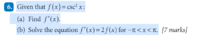# Trigonometric Derivative, Solving f'(x)=2f(x)

#### Subhotosh Khan

##### Super Moderator
Staff member
View attachment 19139
I've solved a, and got f'(x)=-2csc^2(x)cot(x), but now I'm confused as how to solve b.hint for part (b):

if F(y) =e^(n*y)

$$\displaystyle \frac{dF(y)}{dy} = ?$$................. Parts (a) & (b) of the question are not
related.

I probably interpreted the problem [part (b)] incorrectly. See response #3 below.

Last edited: